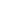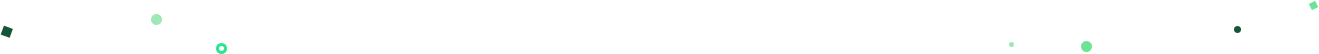## Mode Studio

The Collaborative Data Science Platform# NumPy

NumPy is a scientific computing library for Python. It offers high-level mathematical functions and a multi-dimensional structure (know as `ndarray`) for manipulating large data sets.

While NumPy on its own offers limited functions for data analysis, many other libraries that are key to analysis—such as SciPy, matplotlib, and pandas are heavily dependent on NumPy. SciPy, for instance, offers advanced mathematical functions built on top of NumPy's array data structure, `ndarray`.

NumPy, along with the libraries mentioned above, is a part of the core SciPy stack—a group of tools for scientific computing in Python.

## The NumPy array

NumPy's array (or `ndarray`) is a Python object used for storing data. The main advantage of NumPy over other Python data structures, such as Python's `lists` or pandas' `Series`, is speed at scale. It's most useful when you're creating large matrices with billions of data points.

You don't need a deep understanding of NumPy's array for most analytical tasks—it's more often used for programming—but there are times when it's more efficient than other Python data structures. If you're interested in diving deeper into this issue, check out these resources:

## NumPy features

### Linear algebra

• Computing the eigenvalues of a matrix
• Manipulating linear matrices
• Vectorization

### Statistics# Get our weekly data newsletter

Work-related distractions for every data enthusiast.